### Quantum Computing

The first quiz of this chapter explained how, in a certain sense, a quantum computer isn't really computing at all. It's just allowing the laws of physics to act on a system designed for a very particular purpose.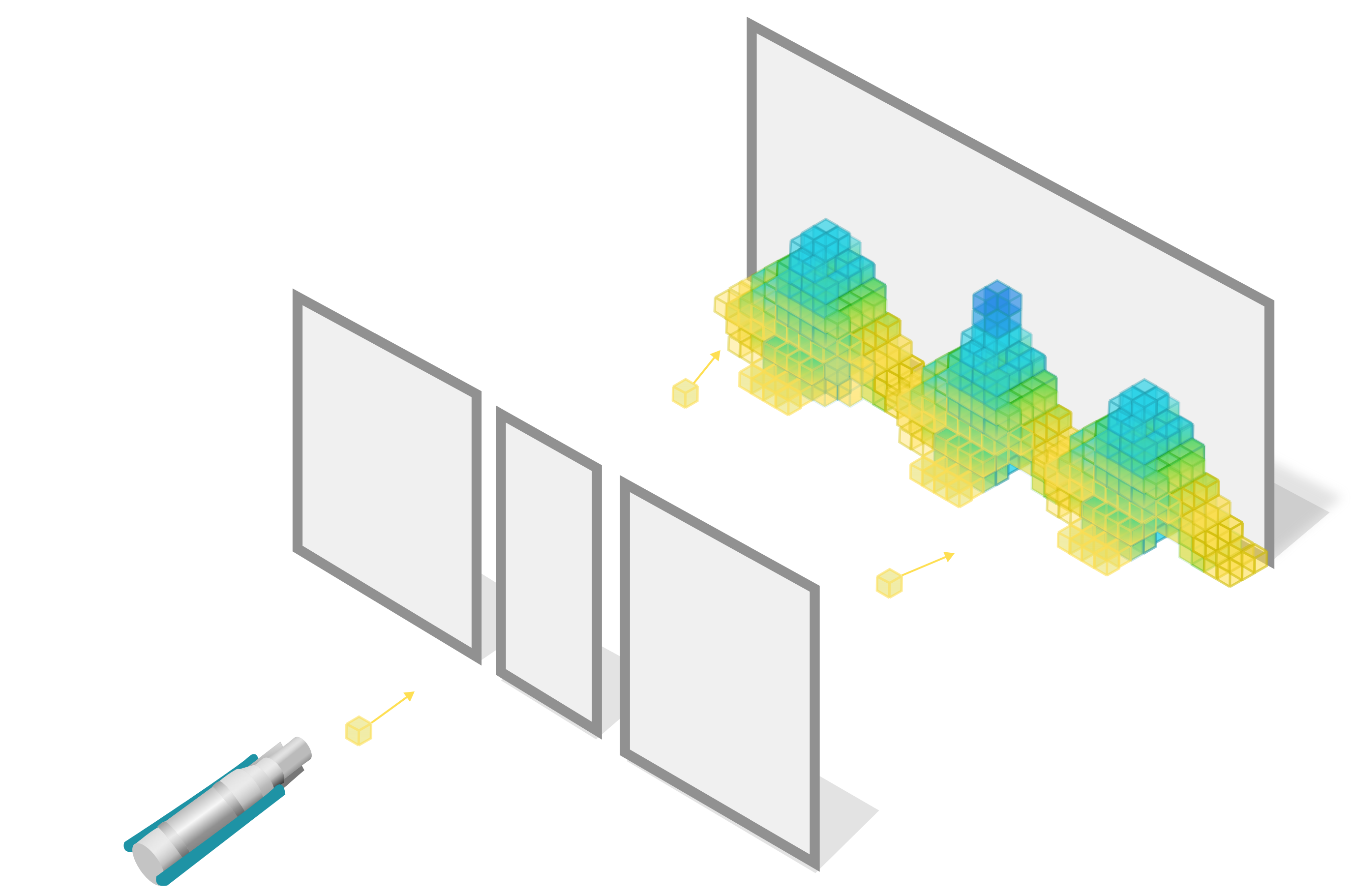The challenge of building a quantum computer is designing components that behave according to the laws of quantum mechanics but can be adapted to solve many problems — not just simulating the path of photons using photons or simulating the electrons in a molecule by studying that molecule.

In this quiz, we'll explore the building blocks of quantum computers and how the unique properties of quantum mechanics can enable a completely new type of information processing.

# Quantum Bits

The first quantum system that we explored in this chapter was an intersecting path of photons and their interactions; but in general a quantum computer can be based on any quantum object that’s easy to measure and manipulate.

As we saw in the last quiz, classical computers perform tasks by using two-state objects called bits as inputs, manipulating them according to some algorithm, then yielding one or more bits as an output that can be measured (e.g. as a voltage).

Much as classical computation uses bits, quantum computation uses qubits, isolated quantum objects that behave quantum mechanically, with two or more states that can be distinguished with a measurement.

# Quantum Bits

A bit is usually stored in a classical computer using a transistor that is either conducting an electric signal (the "high voltage" $1$ state) or not conducting (the "low voltage" $0$ state).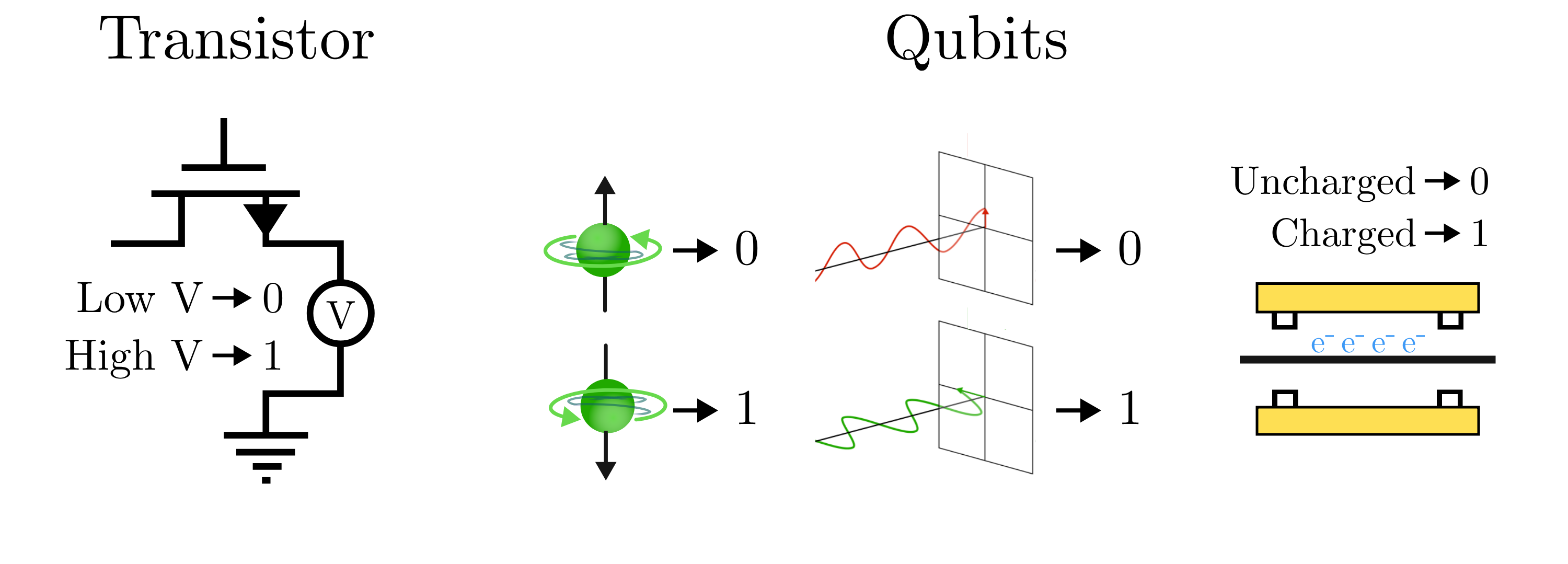A qubit is similar, but it's crafted out of a quantum object — it might be a spinning particle that can point up or down, a photon that can be polarized vertically or horizontally, or a quantum wire that is charged ("on") or uncharged ("off"). Much like a classical bit, the two possible measurements of a qubit have $1$ or $0$ assigned to a certain spin, polarization, or electrical charge.

An isolated qubit with stable assigned states can store a bit of information just like a classical bit. Let's say I'm given a quantum wire, I measure it and find it charged "on" and assign this the state $1$. If I come back some time later, what state should I measure?

Note: To all the seasoned quantum mechanics out there, we are assuming there is no measurement noise affecting our qubit.

# Quantum Bits

We'd like to use a quantum state, say the charge on a tiny quantum wire, to store information. This means we need the measurement to be reproducible, or else it's not a very good information storage medium.

We choose the states useful for computation, which will remain unchanged over many measurements, to represent $1$ and $0$ — and we call these different states the computational states because of this property.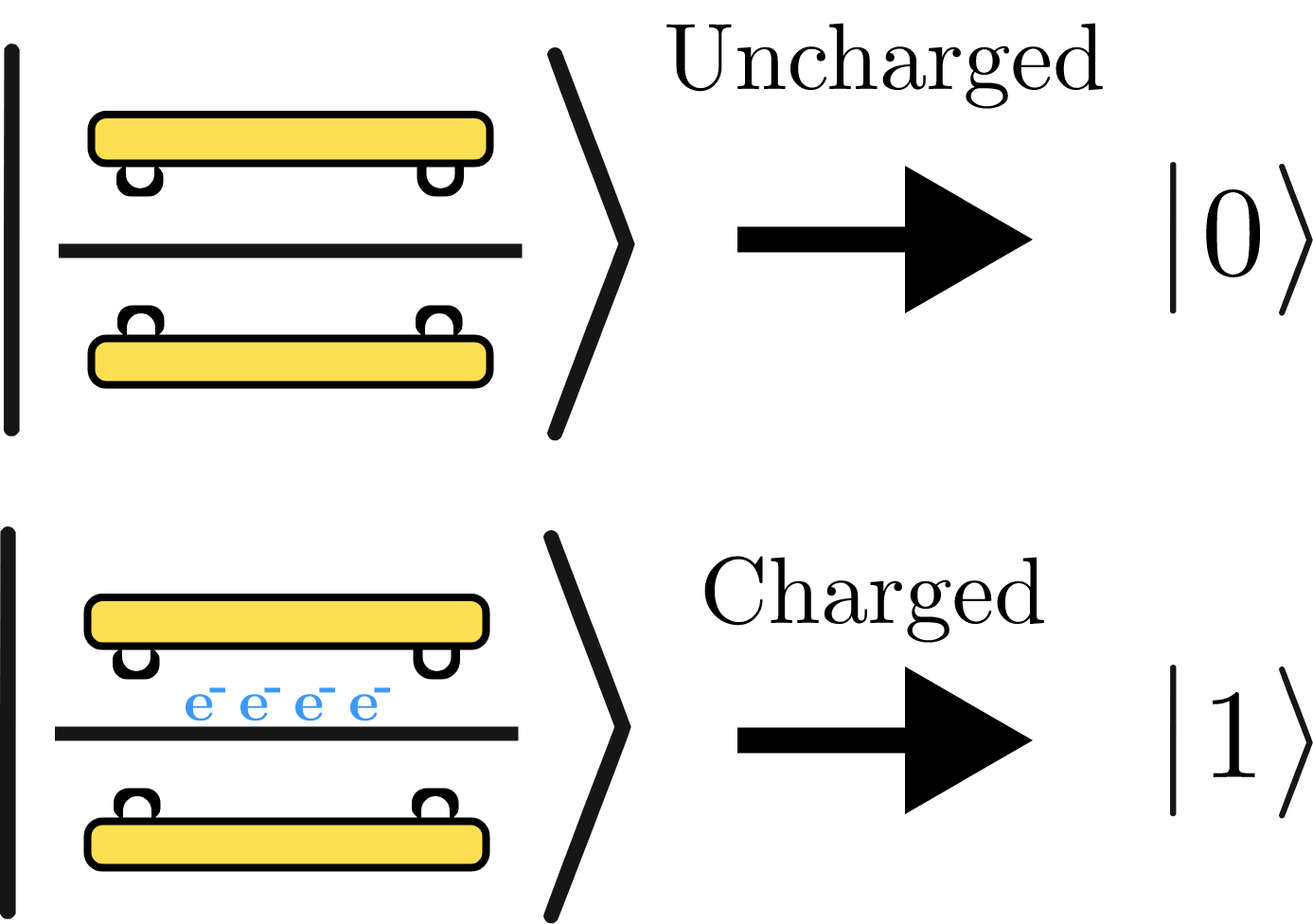No matter the type of qubit you have — a quantum wire, a photon, or a spinning atom — we choose the computational states based on reproducible measurements we can make, and we give them special names because these are quantum states, not classical ones.

The two measurable states of a qubit are called $\ket{0}$ and $\ket{1},$ and we call this way of writing them kets.

# Quantum Bits

So far a qubit is not so different from a classical bit. And indeed, a qubit can do everything a normal bit does! In general, any algorithm that we can run with a classical computer can also be carried out on a quantum computer built from qubits.

While an isolated qubit's state will generally remain unchanged, we can flip a qubit from a $\ket{0}$ to a $\ket{1}$ — sometimes by using a jolt of electricity, and other times by using filter or a flash of light. We can even build up logical circuits like adders out of many quantum bits that operate on input qubits and create output ones that can be measured.

But if you have a nodding familiarity with quantum mechanics, you're probably familiar with its probabilistic nature. Sometimes a state can be in a combination of two states — and you can never be quite sure what you will measure.

Using a different jolt of electricity or even just a polarizing filter that you could buy at a hardware store, it is possible to change a qubit from a state $\ket{0}$ to another new state, a superposition state: $\ket{\psi} = a \ket{0} + b \ket{1}.$ What do you think you'll observe if you try to measure the state of this qubit?

# Quantum Bits

Superposition states are a property of quantum mechanics that truly distinguishes what a qubit is capable of compared to a classical bit. A classical bit can only exist in either a state $1$ or $0$, but a qubit can exist in any combination thereof, even though it will only ever be measured in one or the other: $\ket{\psi} = a \ket{0} + b \ket{1}.$ The coefficients $a$ and $b$ can be any number: not just integers or even real numbers, but any complex number. The relative magnitude of these two coefficients shows how much this superposition state behaves like $\ket{0}$ or $\ket{1}$.

A qubit can be in every possible state from one that has a $100\%$ chance of being measured $\ket{0}$ to one that has a $100\%$ chance of being measured $\ket{1}$. For example, let's say we prepare a qubit into the state $\ket{\psi} = 0.1\ket{0} + 0.995\ket{1}.$ What do you think you'll observe if you try to measure the state of this qubit?

# Quantum Bits

In the most common way of describing a superposition state, the probability of measuring one of the two basis states can be found by finding the relative squared magnitude of each of the coefficients. This probability rule is a fundamental law of nature and one of the primary postulates of quantum mechanics.

We can predict how a state behaves by calculating the relative squared magnitude of the coefficients. Given $0.1\ket{0} + 0.995\ket{1},$ we have \begin{aligned} P\big(\ket{0}\big) &= \frac{0.1^2}{0.1^2 + 0.995^2} = 0.01 = 1 \% \\\\ P\big(\ket{1}\big) &= \frac{0.995^2}{0.1^2 + 0.995^2} = 0.99 = 99 \%. \end{aligned} So, as you may have guessed just by looking at the coefficients, this state has a low likelihood of being measured in the $\ket{0}$ state $(1 \%)$ and a high likelihood of being measured in the $\ket{1}$ state $(99 \%)$.

# Quantum Bits

Since it's only the relative squared magnitude of the coefficients that matters, it follows that any constant multiple of a superposition describes the exact same state: $100 \times \big( 0.1\ket{0} + 0.995\ket{1} \big) = 10\ket{0} + 99.5\ket{1}.$ Try it for yourself, the state with coefficients $10$ and $99.5$ has the same measurement probabilities as one with coefficients $0.1$ and $0.995$.

Since coefficients can be complex numbers, sometimes a phase multiple can appear in a quantum state: $e^{i \frac{\pi}{2}} \big( 0.1\ket{0} + 0.995\ket{1} \big) = 0.1i\ket{0} + 0.995i\ket{1}.$ Will this state have different probabilities than the original state?

# Quantum Bits

Even excluding overall factors or phases, there are a continuum of possible superpositions that a qubit can be prepared in. This is in radical contrast to the two states that a classical bit can occupy.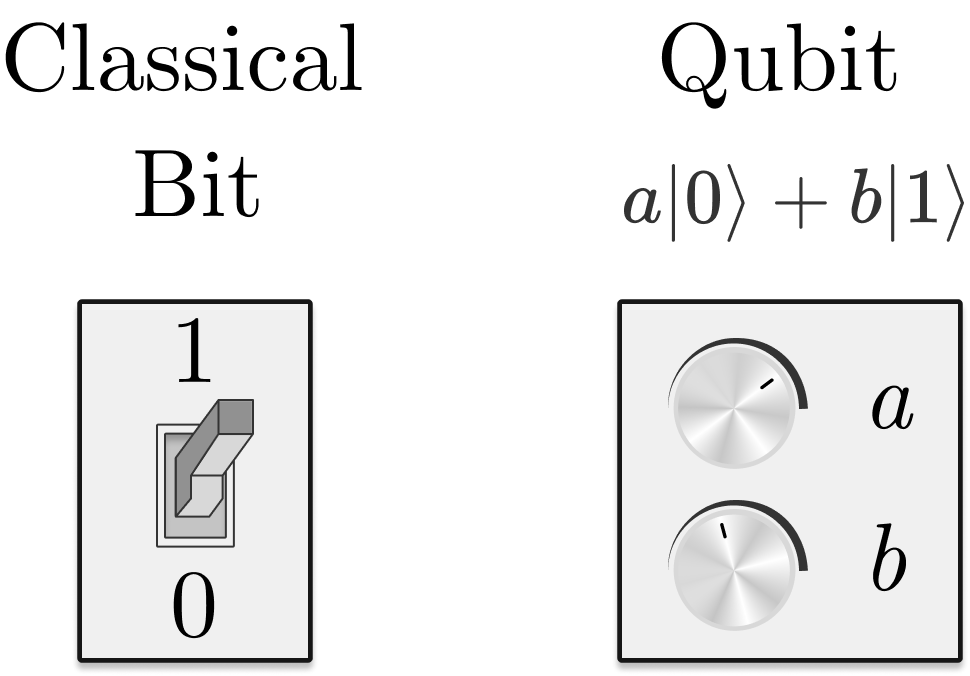But the problem comes in trying to find what the state is through measurements. When you measure a superposition, you will always observe one or the other computational state $\ket{0}$ and $\ket{1}$.

With this in mind, consider the two states we've considered measuring so far: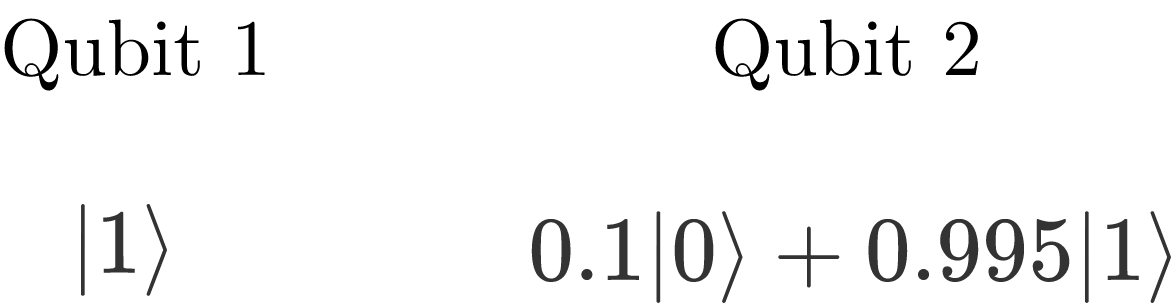Could you always tell the difference between these two qubits based on one measurement?

# Quantum Bits

With a single measurement of qubit $1,$ you have a $100\%$ chance of measuring $\ket{1}$. With a single measurement of qubit $2,$ you have a $99 \%$ chance of the same measurement.

You would need $100$ measurements before you had a good chance of measuring the second qubit in $\ket{0},$ and from your distribution of measurements you could estimate the relative magnitudes in your state.

However, nature doesn't reveal its secrets so easily: a quantum superposition has another trick up its sleeve. After you've measured a qubit and found it in one of its computational states $\ket{0}$ and $\ket{1},$ it will forevermore be found in that same state; measurement changes the state into one of the computational states, and all the other information in that state is lost.

You'll never get your $100$ required measurements, the most you can get is one.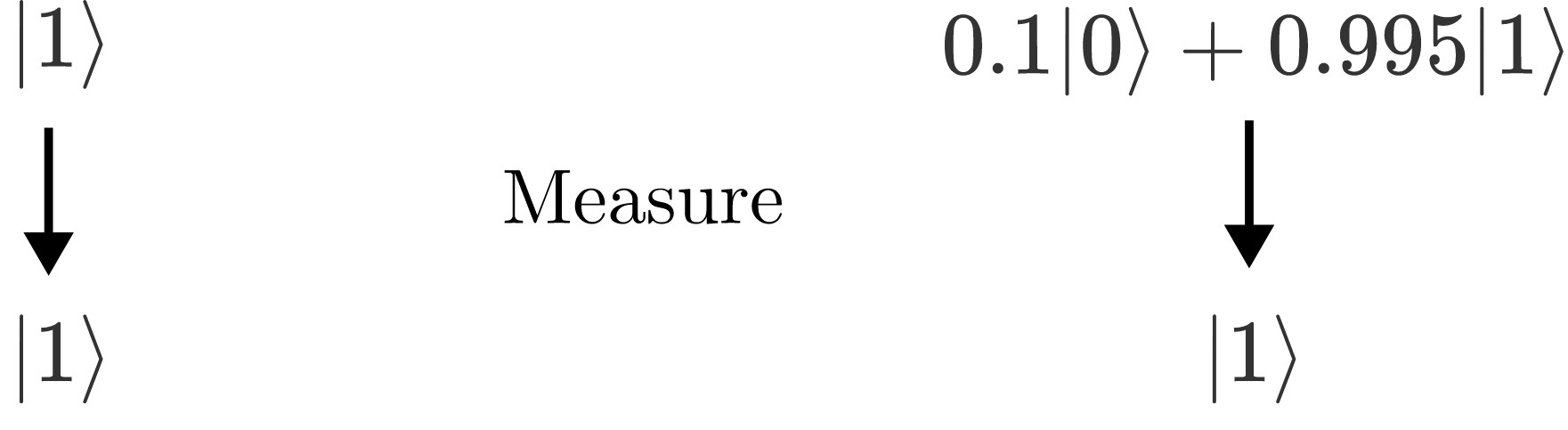# Quantum Bits

The peculiar behavior of quantum states presents the power and the shortcomings of quantum computation: a quantum state's increased complexity compared to a classical bit may be useful for computation, but extracting any of that usefulness through measurement is difficult.Classical computation is performed by switching bits from $0$ to $1$ and back again with a series of logical circuits. The manipulation of qubits during computation uses gates that can modify the coefficients of a superposition, not just flip the state. This vastly increases the information density of a quantum computation, as we'll explore in the next chapter.

However, the challenge is clear: the choreography of quantum computation must all occur without measurement lest the complexity of superposition states be lost.

# Quantum Bits

×